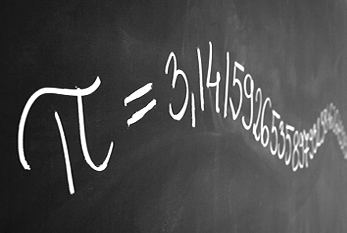# Pi Approximation Day 2022

## July 22, 2022 in the WorldThe first digits of the mathematical constant Pi on a blackboard.

Pi Approximation Day is celebrated on July 22, 2022. Pi Approximation Day is observed on July 22, since the fraction 22?7 is a common approximation of Pi. The number Pi is a mathematical constant that is the ratio of a circle's circumference to its diameter and is approximately equal to 3.14159. It has been represented by a Greek letter since the mid-18th century. Being an irrational number, Pi cannot be expressed exactly as a ratio of any two integers. Consequently, its decimal representation never ends and never settles into a permanent repeating pattern.

A fraction represents a part of a whole or, more generally, any number of equal parts. When spoken in everyday English, a fraction describes how many parts of a certain size there are, for example, one-half, eight-fifths, three-quarters. A common, vulgar, or simple fraction consists of an integer numerator, displayed above a line (or before a slash), and a non-zero integer denominator, displayed below (or after) that line.

An approximation is anything that is similar but not exactly equal to something else. The term can be applied to various properites (e.g. value, quantity, image, description) that are nearly but not exactly correct; similar, but not exactly the same. Although approximation is most often applied to numbers, it is also frequently applied to such things as mathematical functions, shapes, and physical laws. (With material from: Wikipedia)

Where is the event?
Worldwide
World
When is the event?
Friday, the 22nd of July 2022
Only 25 days left!

More dates:

Pi Approximation Day 2022 - Jul 22, 2022 – Copyright © 2022 Cute Calendar